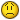sums of range within sumproduct

K

Kayce

It's a bit complicated. I have the following function:

=SUMPRODUCT((INDIRECT(B1)=1)*(INDIRECT(\$A\$1)=2)*C1)

I'd like to calculate a sum of the SUMPRODUCT(...) while varying B1 and C1
together (B1 references the name of a range, C1 references a number), and
then apply the same formula to a new cell that replaces the named range \$A\$1
without having to retype everything. For example:

=SUMPRODUCT((INDIRECT(B1)=1)*(INDIRECT(A1)=2)*C1)+SUMPRODUCT((INDIRECT(B2)=1)*(INDIRECT(A1)=2)*C2)+SUMPRODUCT((INDIRECT(B3)=1)*(INDIRECT(A1)=2)*C3)+SUMPRODUCT((INDIRECT(B4)=1)*(INDIRECT(A1)=2)*C4)

And then that sum repeated for A1-A4. But without having to retype
everything.

S

Spiky

I'm not sure what you are asking. Is this just about how to Copy/
Paste?

There are 2 ways to copy/paste to get various results.

-You can highlight a part of a formula in the Formula Bar, and then
copy/paste it in there over and over so you don't have to retype a
repetitive section. But you'll have to manually change the parts that
do change. Like in your example, the B and C references change, so
you'd have to go back and retype those.

-You can set references to absolute and relative as needed, then copy/
paste one cell to another to have different sums for different ranges.

K

Kayce

Sorry - that wasn't my question.

Let me try and rephrase. I'd like to create a formula to calculate the
summation of some function, say f(x), evaluated at values x=A1 through x=A10.
So, the answer would be something like f(A1)+f(A2)+f(A3)...+f(A10).

Ultimately, I'd like to be able to do the same thing for a function
dependent on two variables, say f(x,y), evaluated at x=A1 through x=A10 and
y=B1 through y=B10, which would evaluate to:
f(A1,B1)+f(A2,B2)+f(A3,B3)...+f(A10,B10).

I'd like to avoid copy/paste, because in reality I'll be doing this
summation for large ranges, and I'd like to avoid making a separate
worksheet, because I'll have many different slight variations on this
equation.

Sorry - I'm coming from Mathematica, which does this in a different way, so
my question might be a bit odd... and confusing.

Thank you!

S

Spiky

Somehow, I don't think our misunderstanding each other is finished.But SUMPRODUCT does sound like the function you want. I think you are
describing array formulas and this function is array by default. The
usual format if you have a variable to check in A, and another
variable in B, and you want to add up numbers in C based on those is:

=SUMPRODUCT(--(\$A\$1:\$A\$10=x)*(\$B\$1:\$B\$10=y),\$C\$1:\$C\$10)

One thing I'm not following is how copy/paste would come up at all.
How are you avoiding that command?

Also, you seem to be saying you want to sum the results of a
sumproduct "lookup". But they are already summed, that's what
sumproduct does when entered the right way. It can also Count,
strangely enough. So, one formula may be all you really need because
of how it works on arrays. Maybe you should show us the form of your
data and then describe what to do to it.

K

Kayce

I think this might be too complicated to explain textually, but I'll try.

The form of my data is I have a bunch of conditions (A-D in this case, but
could be many more), and there are a series of ballots associated with them
that are ranked (there are 10 here, but there could be many more). For
example:

condA 1 1 1 2 1 3 2 1 2 3
condB 2 2 2 1 2 1 1 3 3 2
condC 3 4 3 4 3 2 4 2 4 1
condD 4 3 4 3 4 4 3 4 1 4

And suppose I have another range that shows the minimum (or highest rank) of
each ballot depending on which conditions I choose to include. If all were
included, it would read simply 1 for all ballots, but if I excluded condA,
for example, it would read something like:

min 2 2 2 1 2 1 1 2 1 1

And suppose I also have a weighting factor for each condition that reflects
the weight given to all conditions that are ranked as the "next choice" for
each of the conditions (for example, the second choice of any ballot who
chose condA as first choice is worth 0.5), e.g.:

condA 0.5
condB 0.25
condC 0.1
condD 0.3

I want to be able to find how many ballots in, say, condB (though I want it
found for all conditions) ranked as the second highest choice compared to
condC when condA is eliminated (thus involving the weighting factor and the
minimum)

I think I've found that a formula might work that looks something like:

=SUMPRODUCT(((condA)condD)=1)*((condB)=(min))*E\$1:E\$4)

where "cond_" are named references to their respective ranges ( ie: condA
would be the range of all ballots for condA), min is the range of the minimum
values of each ballot (without ballot A in this case), and E\$1:E\$4 refers to
the 4 cells containing the weighting factors.

I'm not sure semantically how to write all of the ranges so I don't have to
name them all individually and INDIRECT() them all .

Again, this is really hard to describe, so if this kills you, no worries.

Thanks!